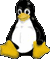# awk Command

awk {'print \$1,\$2,\$3,\$4'}

Print the 1-4 fields only

awk '{if (\$1=="1") print}'

Print the first fields if the value is equal to 1

awk '{if(length(\$4) >= 12) print}'

Print the line if the string length of field 4 is equal or greater than 12

cat fw.log | awk '{for(i=1;i<=NF;i++){ if(\$i~/DST=/) {print \$i} } }' | sort | uniq -c | sort -nr |less

To sort and find the top number of field which including string "DST=" , example :

SRC=1 DST=1 SPT=1 DPT=1
IP=192.168.255.1 SRC=1 DST=1 SPT=1 DPT=1
DST=1 SPT=1 DPT=1
SRC=2 DST=2 SPT=2 DPT=2
DST=3 SPT=3 DPT=3

Result:
3 DST=1
1 DST=2
1 DST=3

cat /var/log/secure* |grep failure |awk '{for(i=1;i<=NF;i++){ if(\$i~/rhost=/) {print \$i} } }' | sort | uniq -c | sort -nr |less

Find the top number which the remote host to access server for "failure" in secure log, actually they are hacker or scanner

cat abc.log |awk '{sum += \$1} END {print sum}'

Sum of the field one for log.

cat abc.log |awk '{print \$NF}'
cat abc.log |awk '{print \$(NF-1)}'

Print the last column or last 2 column

cat ./W3SVC8/u_ex171206.log | cut -d '-' -f5,6,7,8 | awk '{match(\$0,/[0-9]+\.[0-9]+\.[0-9]+\.[0-9]+/); ip = substr(\$0,RSTART,RLENGTH); print ip}' | sort | uniq -c | sort -nr |less

Find the attacker ip / access ip from IIS log via cifs mount (Find IP Addresses with awk)Server is hosted by Alanstudio
Linux Operating System

Recommend screen resolution 1024 x 768 / IE / FireFox